0
0
01. 云栖社区>
2. 博客>
3. 正文

统计02：怎样描绘数据

vamei 2017-04-04 17:15:00 浏览512

群体参数

$$\mu=\frac{1}{N} \sum_{i=0}^N x_i$$

$$\sigma^2=\frac{1}{N} \sum_{i=0}^N (x_i - \mu)^2$$

$$Q_1 = lower\ quartile$$
$$Q_2 = M = median$$
$$Q_3 = upper\ quartile$$
$$IQR = Q_3 - Q_1$$

mean: 172.075924
variance: 102.570849846
standard deviation: 10.1277267857
median: 172.21
lower percentile: 165.31
upper percentile: 178.9025
IQR: 13.5925

import numpy as np

with open("xiangbei_height.txt", "r") as f:

x = list(map(float, lines))
print("mean:", np.mean(x))
print("variance:", np.var(x))
print("standard deviation:", np.std(x))
print("median:", np.median(x))
print("lower percentile:", np.percentile(x, 25))
print("upper percentile:", np.percentile(x, 75))
print("IQR:", np.percentile(x, 75) - np.percentile(x, 25))

数据绘图

饼图

USA        15094025
China      11299967
India       4457784
Japan       4440376
Germany     3099080
Russia      2383402
Brazil      2293954
UK          2260803
France      2217900
Italy       1846950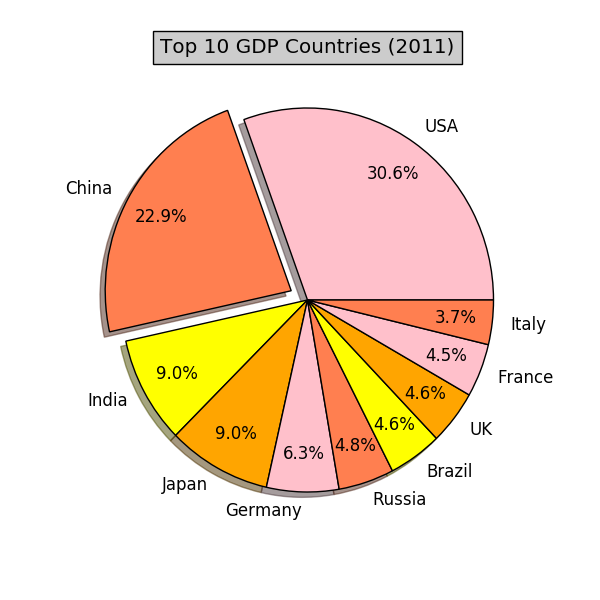import matplotlib.pyplot as plt

# quants: GDP
# labels: country name
labels   = []
quants   = []

with open('major_country_gdp.txt', 'r') as f:
for line in f:
info = line.split()
labels.append(info)
quants.append(float(info))

print(quants)
# make a square figure
plt.figure(1, figsize=(6,6))

# For China, make the piece explode a bit
def explode(label, target='China'):
if label == target:
return 0.1
else:
return 0
expl = list(map(explode,labels))

# Colors used. Recycle if not enough.
colors  = ["pink","coral","yellow","orange"]

# Pie Plot
# autopct: format of "percent" string;
plt.pie(quants,
explode=expl, colors=colors, labels=labels,
plt.title('Top 10 GDP Countries (2011)', bbox={'facecolor':'0.8', 'pad':5})

plt.show()

条形图和直方图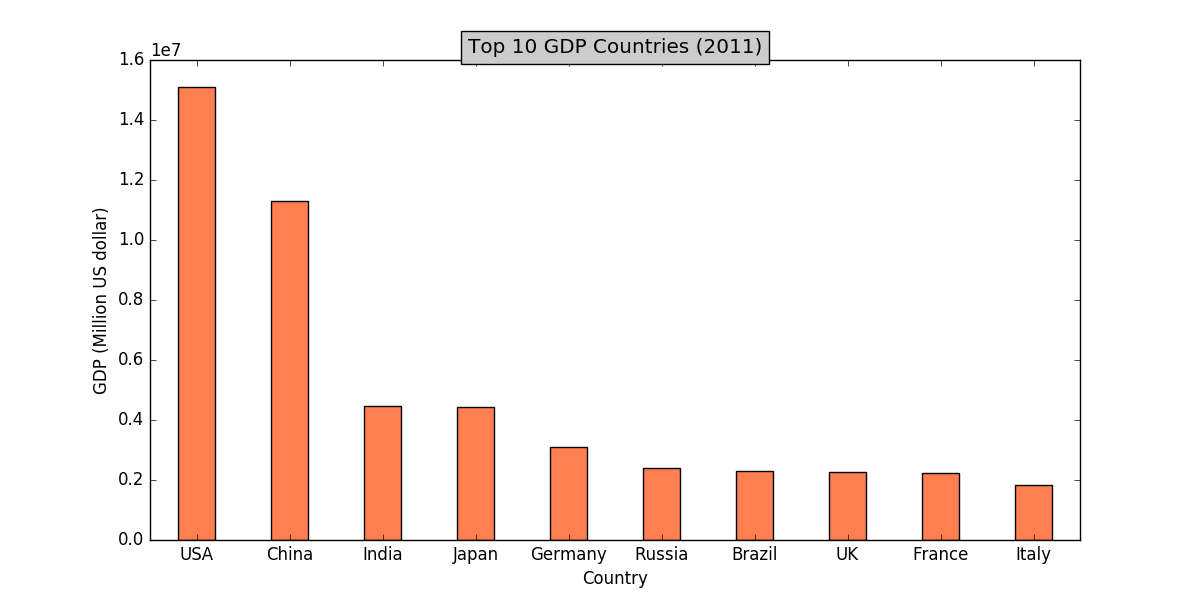import matplotlib.pyplot as plt
import numpy as np

# quants: GDP
# labels: country name
labels   = []
quants   = []

with open('major_country_gdp.txt') as f:
for line in f:
info = line.split()
labels.append(info)
quants.append(float(info))

width = 0.4
ind = np.linspace(0.5,9.5,10)
# make a square figure
fig = plt.figure(1, figsize=(12,6))

# Bar Plot
ax.bar(ind-width/2,quants,width,color='coral')

# Set the ticks on x-axis
ax.set_xticks(ind)
ax.set_xticklabels(labels)
# labels
ax.set_xlabel('Country')
ax.set_ylabel('GDP (Million US dollar)')
# title
ax.set_title('Top 10 GDP Countries (2011)', bbox={'facecolor':'0.8', 'pad':5})
plt.show()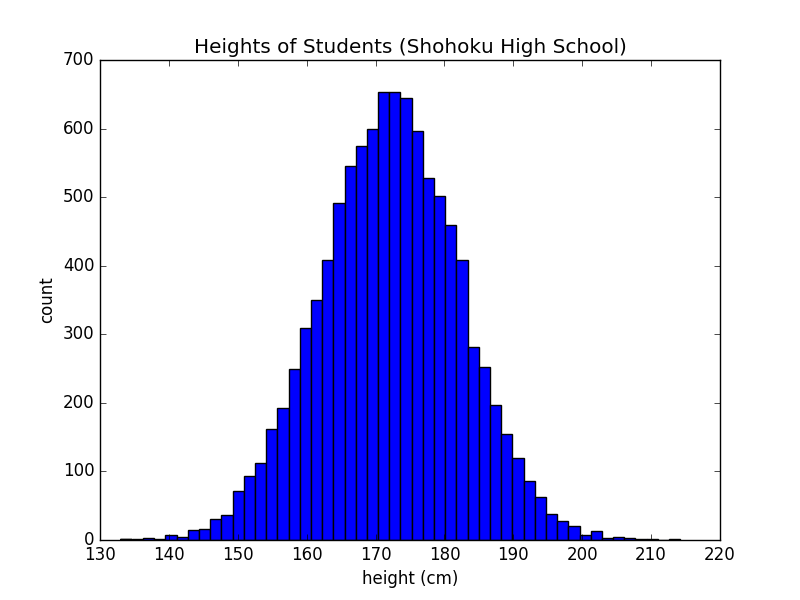import numpy as np
import matplotlib.pyplot as plt

with open("xiangbei_height.txt", "r") as f:

x = list(map(float, lines))

plt.title("Heights of Students (Shohoku High School)")
plt.hist(x, 50)
plt.xlabel("height (cm)")
plt.ylabel("count")
plt.show()

趋势图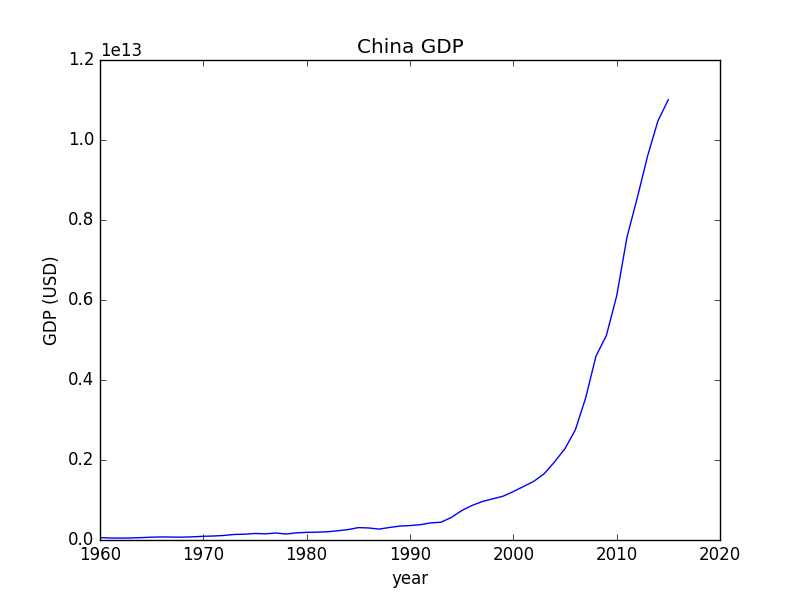import numpy as np
import matplotlib.pyplot as plt

with open("China_GDP.csv", "r") as f:
info = lines.split(",")

# convert data
x = []
y = []

def convert(info_item):
return float(info_item.strip('"'))

for count, info_item in enumerate(info):
try:
y.append(convert(info_item))
x.append(1960 + count)
except ValueError:
print("%s is not a float" % info_item)

# plot
plt.title("China GDP")
plt.plot(x, y)
plt.xlabel("year")
plt.ylabel("GDP (USD)")
plt.show()

散点图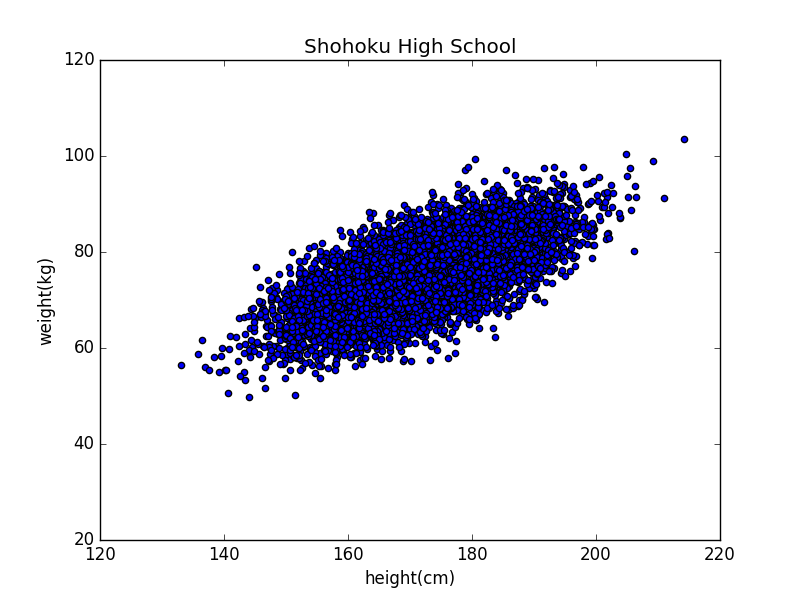import numpy as np
import matplotlib.pyplot as plt

with open(filename) as f:
return np.array(list(map(float, lines)))

plt.scatter(height, weight)

plt.title("Shohoku High School")
plt.xlabel("height(cm)")
plt.ylabel("weight(kg)")
plt.ylim([20, 120])

plt.show()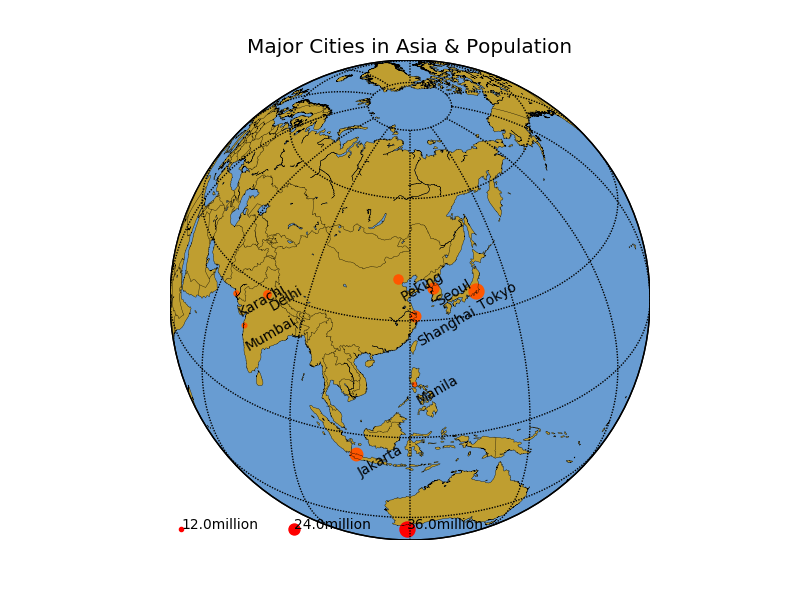Shanghai 23019148  31.23N  121.47E  China
Mumbai   12478447  18.96N  72.82E   India
Karachi  13050000  24.86N  67.01E   Pakistan
Delhi    16314838  28.67N  77.21E   India
Manila   11855975  14.62N  120.97E  Philippines
Seoul    23616000  37.56N  126.99E  Korea(South)
Jakarta  28019545   6.18S  106.83E  Indonesia
Tokyo    35682460  35.67N  139.77E  Japan
Peking   19612368  39.91N  116.39E  China

from mpl_toolkits.basemap import Basemap
import matplotlib.pyplot as plt
import numpy as np

names = []
pops  = []
lats  = []
lons  = []
countries = []

with open("major_city.txt", "r") as f:
for line in f:
info = line.split()
names.append(info)
pops.append(float(info))
lat  = float(info[:-1])
if info[-1] == 'S': lat = -lat
lats.append(lat)
lon  = float(info[:-1])
if info[-1] == 'W': lon = -lon + 360.0
lons.append(lon)
country = info
countries.append(country)

#============================================
# set up map projection with
# use low resolution coastlines.
map = Basemap(projection='ortho',lat_0=35,lon_0=120,resolution='l')

# draw coastlines, country boundaries, fill continents.
map.drawcoastlines(linewidth=0.25)
map.drawcountries(linewidth=0.25)

# draw the edge of the map projection region (the projection limb)
map.drawmapboundary(fill_color='#689CD2')

# draw lat/lon grid lines every 30 degrees.
map.drawmeridians(np.arange(0,360,30))
map.drawparallels(np.arange(-90,90,30))

# Fill continent wit a different color
map.fillcontinents(color='#BF9E30',lake_color='#689CD2',zorder=0)

# compute native map projection coordinates of lat/lon grid.
x, y = map(lons, lats)
max_pop = max(pops)

# Plot each city in a loop.
# Set some parameters
size_factor = 160.0
y_offset    = 15.0
rotation    = 30

adjust_size = lambda k: size_factor*(k-10000000)/max_pop
for i,j,k,name in zip(x,y,pops,names):
plt.text(i,j+y_offset,name,rotation=rotation,fontsize=10)
print(i, j)
examples = [12000000, 24000000, 36000000]

pop = 12000000
plt.text(300000, 300000+y_offset,str(pop/1000000) + "million",rotation=0,fontsize=10)

pop = 24000000
plt.text(3300000, 300000+y_offset,str(pop/1000000) + "million",rotation=0,fontsize=10)

pop = 36000000
plt.text(6300000, 300000+y_offset,str(pop/1000000) + "million",rotation=0,fontsize=10)

plt.title('Major Cities in Asia & Population')
plt.show()

箱形图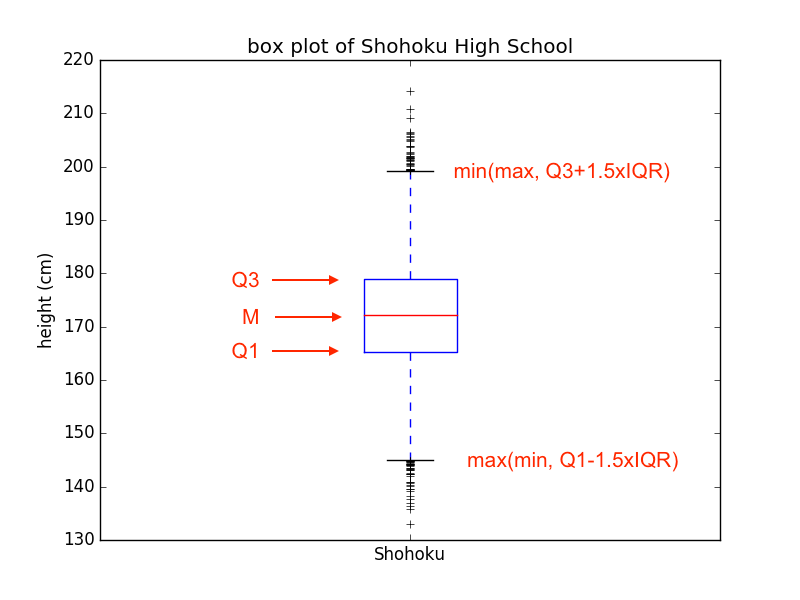import matplotlib.pyplot as plt

with open("xiangbei_height.txt", "r") as f:

x = list(map(float, lines))
plt.boxplot(x)

plt.title("box plot of Shohoku High School")
plt.xticks(, ['Shohoku'])
plt.ylabel("height (cm)")
plt.show()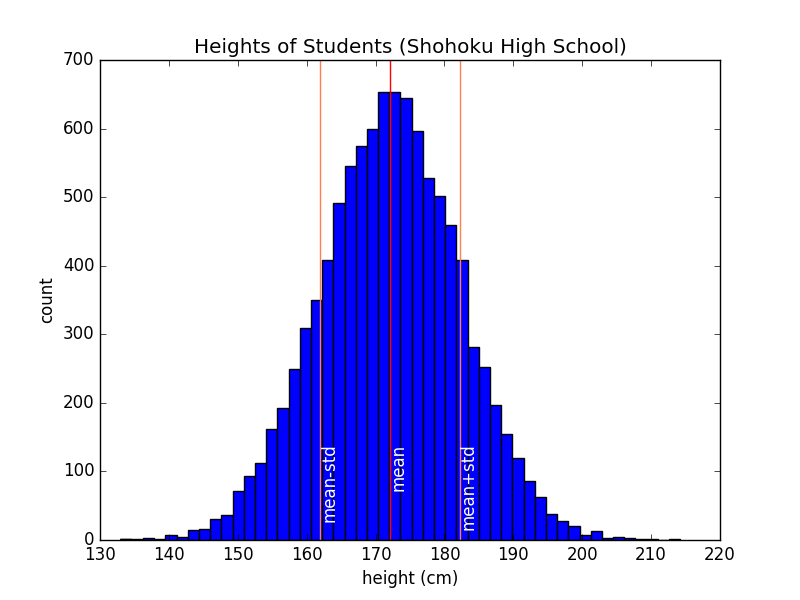import numpy as np
import matplotlib.pyplot as plt

with open("xiangbei_height.txt", "r") as f:

x = list(map(float, lines))

plt.title("Heights of Students (Shohoku High School)")

plt.hist(x, 50)
plt.xlabel("height (cm)")
plt.ylabel("count")

mu  = np.mean(x)
std = np.std(x)

h = 120
text_color = "white"

plt.axvline(x=mu, color="red")
plt.text(mu, h,'mean',rotation=90,color=text_color)

plt.axvline(x=mu-std, color="coral")
plt.text(mu-std, h,'mean-std',rotation=90,color=text_color)

plt.axvline(x=mu+std, color="coral")
plt.text(mu+std, h,'mean+std',rotation=90,color=text_color)

plt.show()

如何画好图

1. 确定目的。尽管在研究过程中，我们会画出大量的数据图，但在展示数据图时，要有所侧重。
2. 在标题中说明一张数据图的主要内容。
3. 标明每一个坐标轴，并标明坐标的刻度和单位。
4. 如果没有坐标轴，需要用图例来说明读数。例如在泡泡图中用图例说明泡泡大小所代表的读数。
5. 在图中标注附加的图像元素，如代表平均值的标示线、代表拟合的虚线曲线等。
6. 备份数据、图像文件和相关代码。

1. 一句话说明画了什么：“这幅图描绘了湘北高中学生身高分布。”
2. 说明坐标轴：“图中横轴代表了身高，纵轴代表了人数。”
3. 说明主要图像元素的含义：“每个竖条对应一定的身高区间。竖条的高度，代表了该身高区间内学生的人数。”
4. 说明次要图像元素的含义：“红线代表了学生的平均身高。”
5. 引导读者深入解读：“可以看到，学生身高大多集中在平均值附近……”

vamei
+ 关注

corcosa 14204人浏览